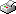Print This Page Termeh Shafie -- doctoral thesis -- Random Multigraphs - Complexity Measures, Probability Models and Statistical Inference Abstract This thesis is concerned with multigraphs and their complexity which is defined and quanti- fied by the distribution of edge multiplicities. Two random multigraph models are considered. The first model is random stub matching (RSM) where the edges are formed by randomly coupling pairs of stubs according to a fixed stub multiplicity sequence. The second model is obtained by independent edge assignments (IEA) according to a common probability distri- bution over the edge sites. Two different methods for obtaining an approximate IEA model from an RSM model are also presented. In Paper I, multigraphs are analyzed with respect to structure and complexity by using entropy and joint information. The main results include formulae for numbers of graphs of different kinds and their complexity. The local and global structure of multigraphs under RSM are analyzed in Paper II. The distribution of multigraphs under RSM is shown to depend on a single complexity statistic. The distributions under RSM and IEA are used for calculations of moments and entropies, and for comparisons by information divergence. The main results include new formulae for local edge probabilities and probability approximation for simplicity of an RSM multigraph. In Paper III, statistical tests of a simple or composite IEA hypothesis are performed using goodness-of-fit measures. The results indicate that even for very small number of edges, the null distributions of the test statistics under IEA have distributions that are well approximated by their asymptotic χ2-distributions. Paper IV contains the multigraph algorithms that are used for numerical calculations in Papers I-III. Key words: multigraph, vertex labeled graph, edge labeled graph, isomorphism, edge multiplicity, simplicity and complexity, entropy, joint information, information divergence, goodness-of-fit. ISBN 978-91-7447-610-1